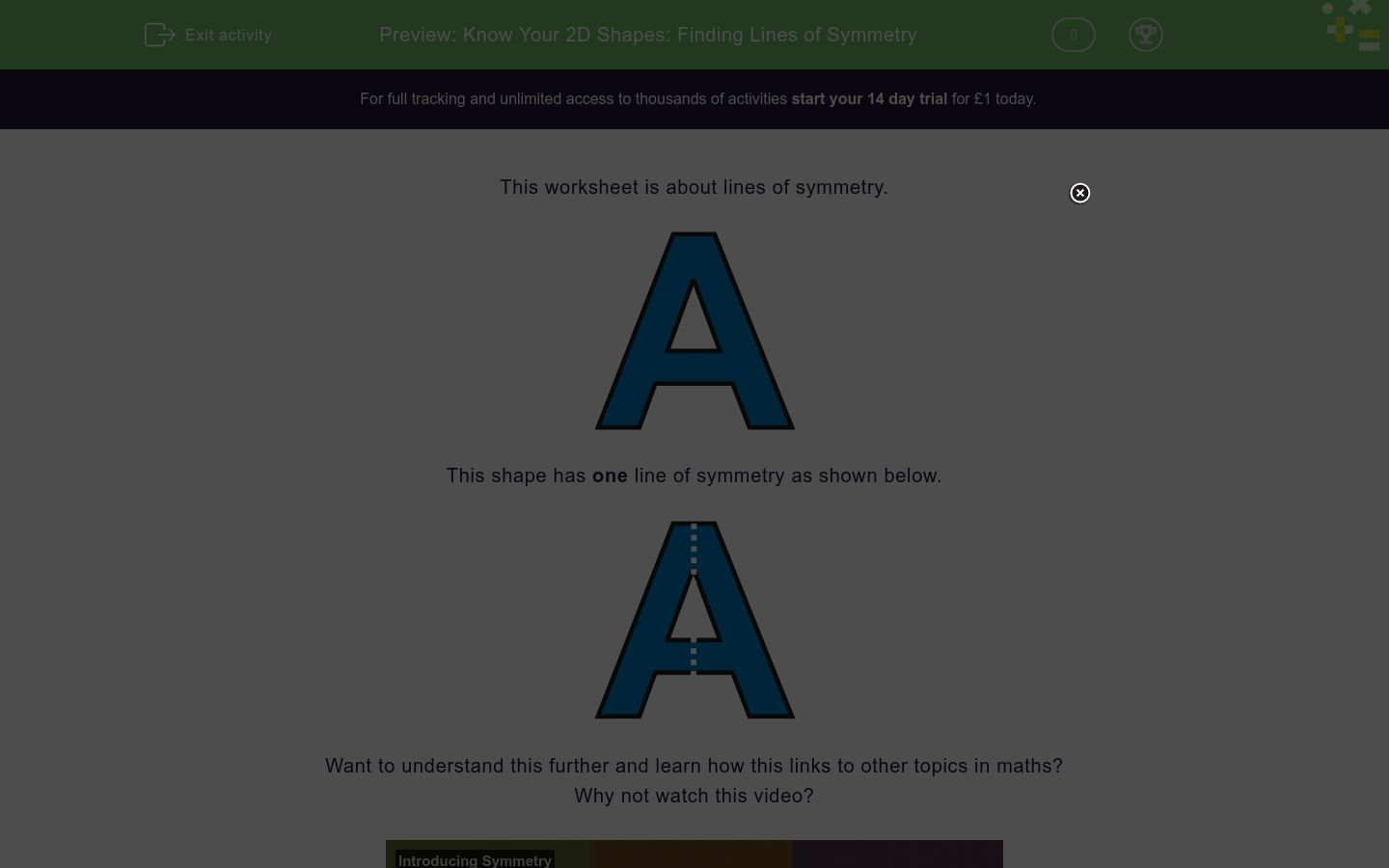# Know Your 2D Shapes: Finding Lines of Symmetry

In this worksheet, students state how many lines of symmetry a shape has.Key stage:  KS 2

Curriculum topic:   Geometry: Properties of Shapes

Curriculum subtopic:   Identify Line Symmetry in 2D Shapes

Difficulty level:### QUESTION 1 of 10

This worksheet is about lines of symmetry.This shape has one line of symmetry as shown below.Want to understand this further and learn how this links to other topics in maths?
Why not watch this video?

How many lines of symmetry can be drawn on this shape?1

2

3

How many lines of symmetry can be drawn on this shape?1

2

3

How many lines of symmetry can be drawn on this shape?1

2

3

How many lines of symmetry can be drawn on this shape?1

2

3

How many lines of symmetry can be drawn on this shape?3

4

5

How many lines of symmetry can be drawn on this shape?1

2

3

How many lines of symmetry can be drawn on this shape?1

3

2

How many lines of symmetry can be drawn on this shape?3

6

4

How many lines of symmetry can be drawn on this shape?8

4

2

How many lines of symmetry can be drawn on this shape?4

0

2

• Question 1

How many lines of symmetry can be drawn on this shape?2
EDDIE SAYS
There are 2 lines of symmetry: down the middle vertically and across the middle horizontally.
• Question 2

How many lines of symmetry can be drawn on this shape?1
EDDIE SAYS
There is 1 line of symmetry: down the middle vertically.
• Question 3

How many lines of symmetry can be drawn on this shape?1
EDDIE SAYS
There is 1 line of symmetry: down the middle vertically.
• Question 4

How many lines of symmetry can be drawn on this shape?1
EDDIE SAYS
There is 1 line of symmetry: down the middle vertically.
• Question 5

How many lines of symmetry can be drawn on this shape?5
EDDIE SAYS
There are 5 lines of symmetry: down the middle vertically and diagonally in 4 different places.
• Question 6

How many lines of symmetry can be drawn on this shape?2
EDDIE SAYS
There are 2 lines of symmetry: down the middle vertically and across the middle horizontally.
• Question 7

How many lines of symmetry can be drawn on this shape?3
EDDIE SAYS
There are 3 lines of symmetry for an equilateral triangle: one starting at each point of the triangle.
• Question 8

How many lines of symmetry can be drawn on this shape?4
EDDIE SAYS
There are 4 lines of symmetry for a square: down the middle vertically, across the middle horizontally and diagonally in two places.
• Question 9

How many lines of symmetry can be drawn on this shape?8
EDDIE SAYS
There are 8 lines of symmetry for an octagon: down the middle vertically, across the middle horizontally and diagonally in six places.
• Question 10

How many lines of symmetry can be drawn on this shape?0
EDDIE SAYS
A parallelogram has no lines of symmetry.
---- OR ----

Sign up for a £1 trial so you can track and measure your child's progress on this activity.

### What is EdPlace?

We're your National Curriculum aligned online education content provider helping each child succeed in English, maths and science from year 1 to GCSE. With an EdPlace account you’ll be able to track and measure progress, helping each child achieve their best. We build confidence and attainment by personalising each child’s learning at a level that suits them.

Get started